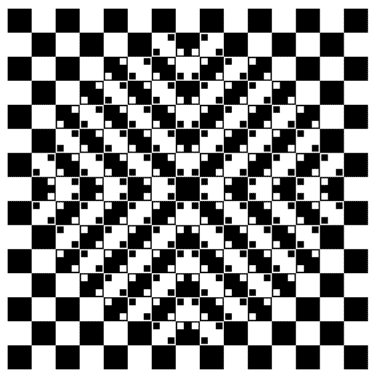### Math brain teasers and optical illusions

Brain Teasers and Optical Illusions:

7 Gallons of Water: (easy)
You have a 3 gallon jug and a 5 gallon jug. You need to measure out exactly 7 gallons of water. How do you do it?

The Missing Dollar: (medium)
Three men in a cafe order a meal the total cost of which is \$15. They each contribute \$5. The waiter takes the money to the chef who recognizes the three as friends and asks the waiter to return \$5 to the men.
The waiter is not only poor at mathematics
but dishonest and instead of going to the trouble of splitting the \$5 between the three he simply gives them \$1 each and pockets the remaining \$2 for himself.
Now, each of the men effectively paid \$4, the total paid is therefore \$12. Add the \$2 in the waiters pocket and this comes to \$14.....where has the other \$1 gone from the original \$15?

A number with an interesting property: (hard)

When I divide it by 2, the remainder is 1.
When I divide it by 3, the remainder is 2.
When I divide it by 4, the remainder is 3.
When I divide it by 5, the remainder is 4.
When I divide it by 6, the remainder is 5.
When I divide it by 7, the remainder is 6.
When I divide it by 8, the remainder is 7.
When I divide it by 9, the remainder is 8.
When I divide it by 10, the remainder is 9.

It's not a small number, but it's not really big, either.
When I looked for a smaller number with this property I couldn't find one.

Can you find it?

Wavy Lines Illusion:
Are these lines straight and parallel or wavy?Look at the chart and say the color not the word:What do you see in this image?Do you see a beautiful woman or a cool jazz horn blower?

Bunny or Duck?
Image Credit: J. Jastrow (1899)